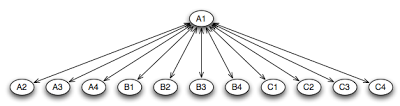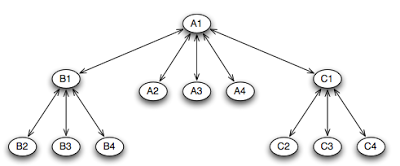# Our Blog

Ongoing observations by End Point Dev people

Sometime you run into a situation where you need to replicate one dataset to many machines in multiple datacenters, with different costs associated with sending to each (either real costs as in bandwidth, or virtual costs as in the amount of time it takes to transmit to each machine). Defining a Slony cluster to handle this is easy, as you can specify the topology and paths taken to replicate any changes.

Basic topology:

• Data center A, with machines A1, A2, A3, and A4.
• Data center B, with machines B1, B2, B3, and B4.
• Data center C, with machines C1, C2, C3, and C4.Figure 1: Non-cascaded slony replication nodes/pathways.

Node A1 is the master, which propagates its changes to all other machines. In the simple setup, A1 would push all of its changes to each node, however if data centers B and C have high costs associated with transfer to the nodes, you end up transferring 4x the data needed for each data center. (We are assuming that traffic on the local subnet at each data center is cheap and fast.)

The basic idea then, is to push the changes only once to each datacenter, and let the “master” machine in the data center push the changes out to the others in the data center. This reduces traffic from the master to each datacenter, plus removes any other associated costs associated with pushing to every node.Figure 2: Cascaded slony replication nodes/pathways

Let’s look at an example configuration:

``````cluster_init.sh:
#!/bin/bash

# admin node definitions and other slony-related information are
# stored in our preamble file.  This will define the \$PREAMBLE
# environment variable that contains basic information common to all
# Slony-related scripts, such as slony cluster name, the nodes
# present, and how to reach them to install slony, etc.

. slony_preamble.sh

slonik <<EOF
\$PREAMBLE

init cluster ( id = 1, comment = 'A1' );

store node (id=2,  comment = 'A2', event node=1);
store node (id=3,  comment = 'A3', event node=1);
store node (id=4,  comment = 'A4', event node=1);
store node (id=5,  comment = 'B1', event node=1);
store node (id=6,  comment = 'B2', event node=1);
store node (id=7,  comment = 'B3', event node=1);
store node (id=8,  comment = 'B4', event node=1);
store node (id=9,  comment = 'C1', event node=1);
store node (id=10, comment = 'C2', event node=1);
store node (id=11, comment = 'C3', event node=1);
store node (id=12, comment = 'C4', event node=1);

# pathways from A1 -> A2, A3, A4 and back
store path (server = 1, client = 2, conninfo = 'dbname=data host=node2.datacenter-a.com');
store path (server = 1, client = 3, conninfo = 'dbname=data host=node3.datacenter-a.com');
store path (server = 1, client = 4, conninfo = 'dbname=data host=node4.datacenter-a.com');
store path (server = 2, client = 1, conninfo = 'dbname=data host=node1.datacenter-a.com');
store path (server = 3, client = 1, conninfo = 'dbname=data host=node1.datacenter-a.com');
store path (server = 4, client = 1, conninfo = 'dbname=data host=node1.datacenter-a.com');

# pathway from A1 -> B1 and back
store path (server = 1, client = 5, conninfo = 'dbname=data host=node1.datacenter-b.com');
store path (server = 5, client = 1, conninfo = 'dbname=data host=node1.datacenter-a.com');

# pathways from B1 -> B2, B3, B4 and back
store path (server = 5, client = 6, conninfo = 'dbname=data host=node2.datacenter-b.com');
store path (server = 5, client = 7, conninfo = 'dbname=data host=node3.datacenter-b.com');
store path (server = 5, client = 8, conninfo = 'dbname=data host=node4.datacenter-b.com');
store path (server = 6, client = 5, conninfo = 'dbname=data host=node1.datacenter-b.com');
store path (server = 7, client = 5, conninfo = 'dbname=data host=node1.datacenter-b.com');
store path (server = 8, client = 5, conninfo = 'dbname=data host=node1.datacenter-b.com');

# pathway from A1 -> C1 and back
store path (server = 1, client = 9, conninfo = 'dbname=data host=node1.datacenter-c.com');
store path (server = 9, client = 1, conninfo = 'dbname=data host=node1.datacenter-a.com');

# pathways from C1 -> C2, C3, C4 and back
store path (server = 9, client = 10, conninfo = 'dbname=data host=node2.datacenter-c.com');
store path (server = 9, client = 11, conninfo = 'dbname=data host=node3.datacenter-c.com');
store path (server = 9, client = 12, conninfo = 'dbname=data host=node4.datacenter-c.com');
store path (server = 10, client = 9, conninfo = 'dbname=data host=node1.datacenter-c.com');
store path (server = 11, client = 9, conninfo = 'dbname=data host=node1.datacenter-c.com');
store path (server = 12, client = 9, conninfo = 'dbname=data host=node1.datacenter-c.com');

EOF
``````

As you can see in the initialization script, we’re defining the basic topology for the cluster. We’re defining each individual node, and the paths that slony will use to communicate events and other status. Since slony needs to communicate status both ways, we need to define the paths for each node’s edge both ways. In particular, we’ve defined pathways from A1 to each of the other A nodes, A1 to B1 and C1, and B1 and C1 to each of their respective nodes.

Now it’s a matter of defining the replication sets and describing the subscriptions for each. We will use something like the following for our script:

``````cluster_define_set1.sh:
#!/bin/bash

# reusing our standard cluster information
. slony_preamble.sh

slonik <<EOF
\$PREAMBLE

create set ( id = 1, origin = 1, comment = 'set 1' );

set add table ( set id = 1, origin = 1, id = 1, fully qualified name = 'public.table1');
set add table ( set id = 1, origin = 1, id = 2, fully qualified name = 'public.table2');
set add table ( set id = 1, origin = 1, id = 3, fully qualified name = 'public.table3');

EOF
``````

Here we’ve defined the tables that we want replicated from A1 to the entire cluster; there is nothing specific to this particular scenario that we need to consider.

``````cluster_subscribe_set1.sh:
#!/bin/bash

# reusing our standard cluster information
. slony_preamble.sh

slonik <<EOF
\$PREAMBLE

# define our forwarding subscriptions (i.e., A1 -> B1, C1)
subscribe set ( id = 1, provider = 1, receiver = 5, forward = yes);
subscribe set ( id = 1, provider = 1, receiver = 9, forward = yes);

# define the subscriptions for each of the datacenter sets
# A1 -> A2, A3, A4
subscribe set ( id = 1, provider = 1, receiver = 2, forward = no);
subscribe set ( id = 1, provider = 1, receiver = 3, forward = no);
subscribe set ( id = 1, provider = 1, receiver = 4, forward = no);

# B1 -> B2, B3, B4
subscribe set ( id = 1, provider = 5, receiver = 6, forward = no);
subscribe set ( id = 1, provider = 5, receiver = 7, forward = no);
subscribe set ( id = 1, provider = 5, receiver = 8, forward = no);

# C1 -> C2, C3, C4
subscribe set ( id = 1, provider = 9, receiver = 10, forward = no);
subscribe set ( id = 1, provider = 9, receiver = 11, forward = no);
subscribe set ( id = 1, provider = 9, receiver = 12, forward = no);

EOF
``````

The key points here are that you specify the provider nodes and the receiver nodes to specify how the particular replication occurs. For the subscription to any cascade point (i.e., B1 and C1), you need to have the ‘forward = yes’ parameter to ensure that the events properly cascade to the sub-nodes. In any of the other nodes’ subscription, you should set ‘forward = no’.

In actual deployment of this setup, you would want to wait for the subscription from A1 -> B1 and A1 -> C1 to complete successfully before subscribing the sub-nodes. Additionally, this solution assumes high availability between nodes and does not address failure of particular machines; in particular, A1, B1, and C1 are key to maintaining the full replication.